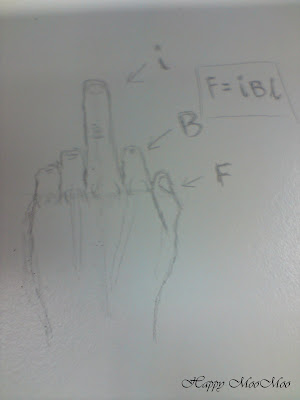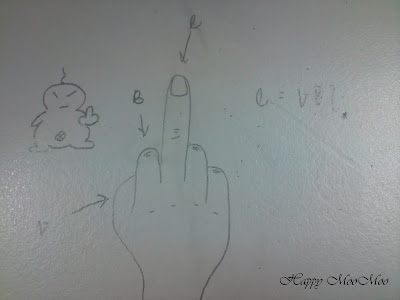## Monday, February 23, 2009

### Hand Rule, Left Hand Rule or Right Hand Rule? (R18)

Uni Life Chapter 12

Let's learn some hand rules today ^^. First question. Which hand rule do you use to find the force on a current in a magnetic field? The Left Hand Rule or the Right Hand Rule?

The answer is the Left Hand Rule!An illustration from Dr. Mutasim's notesAn illustration by a.k.a. Stripper

Lets move on to the second question. Which hand rule do you use to find the voltage induced in a moving conductor? The Left Hand Rule or the Right Hand Rule?

The answer is obviously the Right Hand Rule.. cause the answer to the first question is the Left Hand Rule.. so the answer for this should be the Right Hand Rule.. right? XDAn illustration from Dr. Mutasim's notesAn illustration by me XD

I know I know.. My drawing su*** LOL..